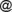# Issue:Conditional intuitionistic fuzzy probability and martingale convergence theorem using IF-probability

 shortcut http://ifigenia.org/wiki/issue:nifs/26/3/13-21
Title of paper: Conditional intuitionistic fuzzy probability and martingale convergence theorem using IF-probability
Author(s):
 Katarína Čunderlíková Mathematical Institute, Slovak Academy of Sciences, Dumbierska 1, Banska Bystrica, Slovakia cunderlikova.lendelovagmail.com
Published in: Notes on Intuitionistic Fuzzy Sets, Volume 26 (2020), Number 3, pages 13–21
DOI: https://doi.org/10.7546/nifs.2020.26.3.13-21
Abstract: The aim of this paper is to formulate the conditional intuitionistic fuzzy probability and a version of martingale convergence theorem with respect an intuitionistic fuzzy probability. Since the intuitionistic fuzzy probability can be decomposed to two intuitionistic fuzzy states, we can use the results holding for intuitionistic fuzzy states.
Keywords: Intuitionistic fuzzy event, Intuitionistic fuzzy observable, Intuitionistic fuzzy state, Intuitionistic fuzzy probability, Product, Conditional intuitionistic fuzzy probability, Martingale convergence theorem.
AMS Classification: 03B52, 60A86, 60G48.
References:
1. Atanassov, K. (1999). Intuitionistic Fuzzy Sets: Theory and Applications, Springer Physica Verlag, Heindelberg.
2. Atanassov, K. T. (2012). On Intuitionistic Fuzzy Sets, Springer, Berlin.
3. Čunderlíková, K. (2019). Intuitionistic fuzzy probability and almost everywhere convergence, Proceedings of IWIFSGN 2019, 24-25 October 2019, Warsaw, Poland (accepted).
4. Čunderlíková, K. (2020). Martingale convergence theorem for the conditional intuitionistic fuzzy probability, Mathematics, 8 (10), 1707, 1–10.
5. Grzegorzewski, P., & Mrowka, E. (2002). Probability of intuitionistic fuzzy events, Soft Methods in Probability, Statistics and Data Analysis, P. Grzegorzewski et al. eds., Physica Verlag, New York, 105–115.
6. Lendelová, K. (2006). Conditional IF-probability, Advances in Soft Computing: Soft Methods for Integrated Uncertainty Modelling, 275–283.
7. Lendelová, K., & Riecan, B. (2004). Weak law of large numbers for IF-events, Current Issues in Data and Knowledge Engineering, Bernard De Baets et al. eds., EXIT, Warszawa, 309–314.
8. Riecan, B. (2003). A descriptive definition of the probability on intuitionistic fuzzy sets, EUSFLAT ’2003, M. Wagenecht, R. Hampet eds., Zittau-Goerlitz Univ. Appl. Sci., 263– 266.
9. Riecan, B. (2005). On the probability on IF-sets and MV-algebras, Notes on Intuitionistic Fuzzy Sets, 11 (6), 21–25.
10. Riecan, B. (2006). On a problem of Radko Mesiar: general form of IF-probabilities, Fuzzy Sets and Systems, 152, 1485–1490.
11. Riecan, B. (2006) On the probability and random variables on IF events, Applied Artificial Intelligence, Proc. 7th FLINS Conf. Genova, D. Ruan et al. eds., 138–145.
12. Riecan, B. (2007). Probability theory on intuitionistic fuzzy events, Algebraic and Proof-theoretic aspects of Non-classical Logics, Papers in honour of Daniele Mundici’s 60th birthday, Lecture Notes in Computer Science, Vol. 4460.
13. Riecan, B. (2012). Analysis of fuzzy logic models, Intelligent Systems (V. Koleshko ed.), INTECH, 219–244.
14. Riecan, B., & Neubrunn, T. (1997). Integral, Measure, and Ordering, Kluwer Academic Publishers, Dordrecht and Ister Science, Bratislava.
Citations:

The list of publications, citing this article may be empty or incomplete. If you can provide relevant data, please, write on the talk page.